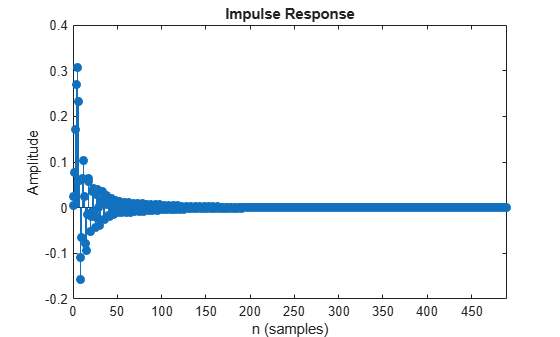# impz

Impulse response of discrete-time filter System object

## Syntax

``````[impResp,t] = impz(sysobj)``````
``````[impResp,t] = impz(sysobj,n)``````
``````[impResp,t] = impz(sysobj,n,fs)``````
``````[impResp,t] = impz(sysobj,[],fs)``````
``````[impResp,t] = impz(sysobj,'Arithmetic',arithType)``````
``impz(sysobj)``

## Description

example

``````[impResp,t] = impz(sysobj)``` computes the impulse response of the filter System object™ and returns the response in column vector `impResp`, and a vector of times (or sample intervals) in `t`, where `t = [0 1 2 ...k-1]'`. `k` is the number of filter coefficients.```
``````[impResp,t] = impz(sysobj,n)``` computes the impulse response at `floor(n)` one-second intervals. The time vector `t` equals `(0:floor(n)-1)'`.```
``````[impResp,t] = impz(sysobj,n,fs)``` computes the impulse response at `floor(n)` 1/`fs`-second intervals. The time vector `t` equals `(0:floor(n)-1)'/fs`.```
``````[impResp,t] = impz(sysobj,[],fs)``` computes the impulse response at `k` 1/`fs`-second intervals. `k` is the number of filter coefficients. The time vector `t` equals `(0:k-1)'/fs`.```
``````[impResp,t] = impz(sysobj,'Arithmetic',arithType)``` computes the impulse response based on the arithmetic specified in `arithType`, using either of the previous syntaxes.```
````impz(sysobj)` uses `fvtool` to plot the impulse response of the filter System object `sysobj`.You can use `impz` for both real and complex filters. When you omit the output arguments, `impz` plots only the real part of the impulse response.For more input options, refer to `impz` in Signal Processing Toolbox™.```

## Examples

collapse all

Create a discrete-time filter for a fourth-order, lowpass elliptic filter with a cutoff frequency of 0.4 times the Nyquist frequency. Use a second-order sections structure to resist quantization errors. Plot the first 50 samples of the impulse response, along with the reference impulse response.

`d = fdesign.lowpass(.4,.5,1,80);`

Create a design object for the prototype filter. Use `ellip` to design a minimum order discrete-time SOS filter.

`sosFilt = design(d,'ellip','Systemobject',true)`
```sosFilt = dsp.SOSFilter with properties: Structure: 'Direct form II' CoefficientSource: 'Property' Numerator: [4x3 double] Denominator: [4x3 double] HasScaleValues: true ScaleValues: [0.7277 0.8290 1.3494 0.0048 1] Show all properties ```

Plot the impulse response.

`impz(sosFilt);`## Input Arguments

collapse all

Length of the impulse response vector, specified as a positive integer.

Data Types: `single` | `double` | `int8` | `int16` | `int32` | `int64` | `uint8` | `uint16` | `uint32` | `uint64`

Sampling frequency used in computing the impulse response, specified as a positive scalar.

Data Types: `single` | `double` | `int8` | `int16` | `int32` | `int64` | `uint8` | `uint16` | `uint32` | `uint64`

Arithmetic used in the filter analysis, specified as `'double'`, `'single'`, or `'Fixed'`. When the arithmetic input is not specified and the filter System object is unlocked, the analysis tool assumes a double-precision filter. When the arithmetic input is not specified and the System object is locked, the function performs the analysis based on the data type of the locked input.

The `'Fixed'` value applies to filter System objects with fixed-point properties only.

When the `'Arithmetic'` input argument is specified as `'Fixed'` and the filter object has the data type of the coefficients set to `'Same word length as input'`, the arithmetic analysis depends on whether the System object is unlocked or locked.

• unlocked –– The analysis object function cannot determine the coefficients data type. The function assumes that the coefficients data type is signed, has a 16-bit word length, and is auto scaled. The function performs fixed-point analysis based on this assumption.

• locked –– When the input data type is `'double'` or `'single'`, the analysis object function cannot determine the coefficients data type. The function assumes that the data type of the coefficients is signed, has a 16-bit word length, and is auto scaled. The function performs fixed-point analysis based on this assumption.

To check if the System object is locked or unlocked, use the `isLocked` function.

When the arithmetic input is specified as `'Fixed'` and the filter object has the data type of the coefficients set to a custom numeric type, the object function performs fixed-point analysis based on the custom numeric data type.

## Output Arguments

collapse all

Impulse response, returned as an `n`-element vector. If `n` is not specified, the length of the impulse response vector equals the number of coefficients in the filter.

Data Types: `double`

Time vector of length `n`, in seconds. `t` consists of `n` equally spaced points in the range `(0:floor(n)-1)'/fs`. If `n` is not specified, the function uses the number of coefficients of the filter.

Data Types: `double`

## Version History

Introduced in R2011a